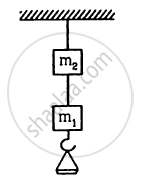Department of Pre-University Education, KarnatakaPUC Karnataka Science Class 11

# The Two Wires Shown in Figure Are Made of the Same Material Which Has a Breaking Stress of 8 × 108 N M−2. Repeat the Above Part If M1 = 10 Kg and M2 = 36 Kg. - Physics

Short Note

The two wires shown in figure are made of the same material which has a breaking stress of 8 × 108 N m−2. The area of cross section of the upper wire is 0.006 cm2 and that of the lower wire is 0.003 cm2. The mass m1 = 10 kg, m2 = 20 kg and the hanger is light.   Repeat the above part if m1 = 10 kg and m2 = 36 kg.#### Solution

$\text{If m}_1 = 10 \text{ kg and m}_2 = 36 \text{ kg }$
Tension in lower wire  $\text{T}_\text{l} = \text{m}_1 \text{ g + w}$
Here: g is the acceleration due to gravity
∴ Stress in lower wire:
$\Rightarrow \frac{\text{T}_\text{l}}{\text{A}_\text{l}} = \frac{\text{m}_1\text{ g + w}}{\text{A}_\text{l}} = 8 \times {10}^5$
$\Rightarrow \text{ w = 140 N }$
Now, tension in upper wire
$\text{T}_2 = \text{m}_1 \text{ g + m}_2 \text{ g + w }$
∴ Stress in upper wire:
$\Rightarrow \frac{\text{T}_\text{u}}{\text{A}_\text{u}} = \frac{\text{m}_2 \text{g + m}_1 \text{g + w}}{\text{A}_\text{u}} = 8 \times {10}^5$
$\Rightarrow \text{w = 20 N}$

For the same breaking stress, the maximum load that can be put is 20 N or 2 kg. The upper wire will break first if the load is increased.

Concept: Elastic Moduli - Determination of Young’s Modulus of the Material of a Wire
Is there an error in this question or solution?

#### APPEARS IN

HC Verma Class 11, Class 12 Concepts of Physics Vol. 1
Chapter 14 Some Mechanical Properties of Matter
Q 6.2 | Page 300